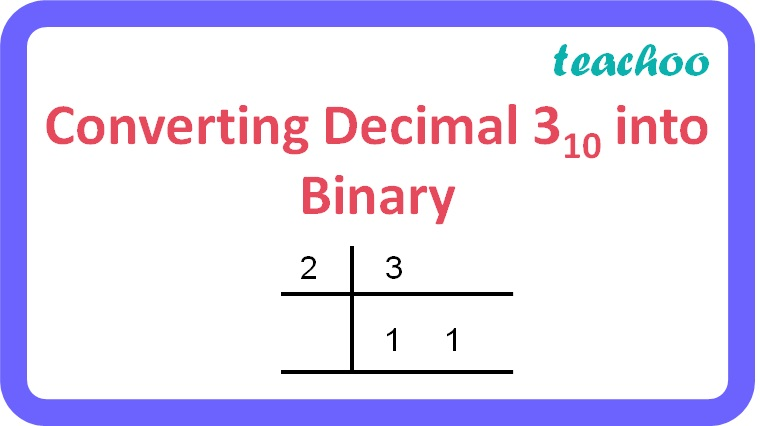MCQ questions (1 mark each)

Computer Science - Class 11
Chapter 2 Class 11 - Encoding Schemes and Number System

## (d) 1010 2

3 10 is a decimal number.

To convert a decimal number to its binary equivalent:

• Repeatedly divide the decimal number by 2 until the quotient becomes 1 and record all the remainders.
• The remainders should be written bottom to upwards to get the binary equivalent of the decimal number.Therefore, 3 10 = 11 2 = 0011 2

So, the correct answer is (b).

Learn in your speed, with individual attention - Teachoo Maths 1-on-1 Class